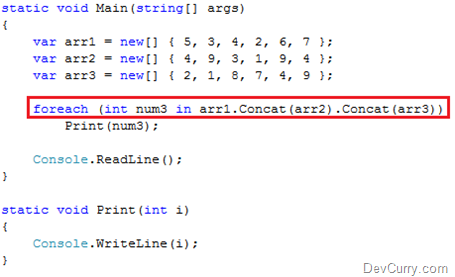# Loop Multiple Arrays in C# – Short way

 Tweet

Let us see a trick to loop multiple arrays in C#. Consider the following program:

`static void Main(string[] args){    var arr1 = new[] { 5, 3, 4, 2, 6, 7 };    var arr2 = new[] { 4, 9, 3, 1, 9, 4 };    var arr3 = new[] { 2, 1, 8, 7, 4, 9 };    foreach (int num in arr1)        Print(num);    foreach (int num1 in arr2)        Print(num1);    foreach (int num2 in arr3)        Print(num2);}static void Print(int i){    Console.WriteLine(i);}}`

As you can see, we are using three loops to print the contents of the array. Using the LINQ Concat operator, we can shorten the code by reducing three loops into just one, as shown belowIf you liked this tip, check some more LINQ Tips